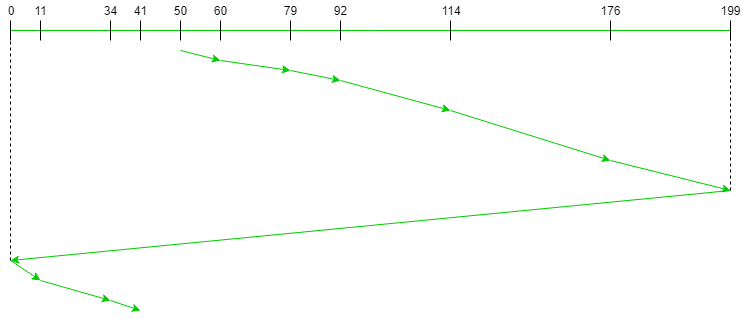# C-SCAN Disk Scheduling Algorithm

Prerequisite: Disk Scheduling Algorithms and SCAN Disk Scheduling Algorithm

Given an array of disk track numbers and initial head position, our task is to find the total number of seek operations done to access all the requested tracks if C-SCAN disk scheduling algorithm is used.

What is C-SCAN (Circular Elevator) Disk Scheduling Algorithm?
Circular SCAN (C-SCAN) scheduling algorithm is a modified version of SCAN disk scheduling algorithm that deals with the inefficiency of SCAN algorithm by servicing the requests more uniformly. Like SCAN (Elevator Algorithm) C-SCAN moves the head from one end servicing all the requests to the other end. However, as soon as the head reaches the other end, it immediately returns to the beginning of the disk without servicing any requests on the return trip (see chart below) and starts servicing again once reaches the beginning. This is also known as the “Circular Elevator Algorithm” as it essentially treats the cylinders as a circular list that wraps around from the final cylinder to the first one.

Algorithm:

1. Let Request array represents an array storing indexes of tracks that have been requested in ascending order of their time of arrival. ‘head’ is the position of disk head.
2. The head services only in the right direction from 0 to size of the disk.
3. While moving in the left direction do not service any of the tracks.
4. When we reach at the beginning(left end) reverse the direction.
5. While moving in right direction it services all tracks one by one.
6. While moving in right direction calculate the absolute distance of the track from the head.
7. Increment the total seek count with this distance.
8. Currently serviced track position now becomes the new head position.
9. Go to step 6 until we reach at right end of the disk.
10. If we reach at the right end of the disk reverse the direction and go to step 3 until all tracks in request array have not been serviced.

Examples:

```Input:
Request sequence = {176, 79, 34, 60, 92, 11, 41, 114}

Output:
Total number of seek operations = 190
Seek Sequence is
60
79
92
114
176
199
0
11
34
41
```

The following chart shows the sequence in which requested tracks are serviced using SCAN.Therefore, the total seek count is calculated as:

```= (60-50)+(79-60)+(92-79)
+(114-92)+(176-114)+(199-176)+(199-0)
+(11-0)+(34-11)+(41-34) ```

Implementation:
Implementation of C-SCAN algorithm is given below.

Note:
The distance variable is used to store the absolute distance between the head and current track position. disk_size is the size of the disk. Vectors left and right stores all the request tracks on the left-hand side and the right-hand side of the initial head position respectively.

 `#include ` `using namespace std; ` ` `  `// Code by Vikram Chaurasia ` ` `  `// C++ program to demonstrate ` `// C-SCAN Disk Scheduling algorithm ` ` `  `int size = 8; ` `int disk_size = 200; ` ` `  `void CSCAN(int arr[], int head) ` `{ ` `    ``int seek_count = 0; ` `    ``int distance, cur_track; ` `    ``vector left, right; ` `    ``vector seek_sequence; ` ` `  `    ``// appending end values ` `    ``// which has to be visited ` `    ``// before reversing the direction ` `    ``left.push_back(0); ` `    ``right.push_back(disk_size - 1); ` ` `  `    ``// tracks on the left of the ` `    ``// head will be serviced when ` `    ``// once the head comes back ` `    ``// to the beggining (left end). ` `    ``for (int i = 0; i < size; i++) { ` `        ``if (arr[i] < head) ` `            ``left.push_back(arr[i]); ` `        ``if (arr[i] > head) ` `            ``right.push_back(arr[i]); ` `    ``} ` ` `  `    ``// sorting left and right vectors ` `    ``std::sort(left.begin(), left.end()); ` `    ``std::sort(right.begin(), right.end()); ` ` `  `    ``// first service the requests ` `    ``// on the right side of the ` `    ``// head. ` `    ``for (int i = 0; i < right.size(); i++) { ` `        ``cur_track = right[i]; ` `        ``// appending current track to seek sequence ` `        ``seek_sequence.push_back(cur_track); ` ` `  `        ``// calculate absolute distance ` `        ``distance = abs(cur_track - head); ` ` `  `        ``// increase the total count ` `        ``seek_count += distance; ` ` `  `        ``// accessed track is now new head ` `        ``head = cur_track; ` `    ``} ` ` `  `    ``// once reached the right end ` `    ``// jump to the beggining. ` `    ``head = 0; ` ` `  `    ``// Now service the requests again ` `    ``// which are left. ` `    ``for (int i = 0; i < left.size(); i++) { ` `        ``cur_track = left[i]; ` ` `  `        ``// appending current track to seek sequence ` `        ``seek_sequence.push_back(cur_track); ` ` `  `        ``// calculate absolute distance ` `        ``distance = abs(cur_track - head); ` ` `  `        ``// increase the total count ` `        ``seek_count += distance; ` ` `  `        ``// accessed track is now the new head ` `        ``head = cur_track; ` `    ``} ` ` `  `    ``cout << "Total number of seek operations = " ` `         ``<< seek_count << endl; ` ` `  `    ``cout << "Seek Sequence is" << endl; ` ` `  `    ``for (int i = 0; i < seek_sequence.size(); i++) { ` `        ``cout << seek_sequence[i] << endl; ` `    ``} ` `} ` ` `  `// Driver code ` `int main() ` `{ ` ` `  `    ``// request array ` `    ``int arr[size] = { 176, 79, 34, 60, ` `                      ``92, 11, 41, 114 }; ` `    ``int head = 50; ` ` `  `    ``cout << "Initial position of head: " << head << endl; ` `    ``CSCAN(arr, head); ` ` `  `    ``return 0; ` `} `

```Output:
Total number of seek operations = 190
Seek Sequence is
60
79
92
114
176
199
0
11
34
41
```My Personal Notes arrow_drop_upCheck out this Author's contributed articles.

If you like GeeksforGeeks and would like to contribute, you can also write an article using contribute.geeksforgeeks.org or mail your article to contribute@geeksforgeeks.org. See your article appearing on the GeeksforGeeks main page and help other Geeks.

Please Improve this article if you find anything incorrect by clicking on the "Improve Article" button below.

Improved By : wilsonmun